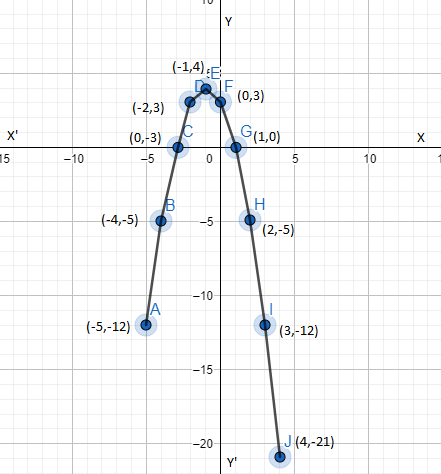QUESTION

# Draw the graphs of the quadratic polynomial, $f(x)=3-2x-{{x}^{2}}$.

Hint: Put $f(x)=y$. Thus give x various values and get the coordinates (x, y) to plot the graph. After plotting the points, join the points with a smooth free hand curve and identify the curve that we have obtained.
Given us is the quadratic polynomial $f(x)=3-2x-{{x}^{2}}$.
Let us put $f(x)=y$.
$\therefore y=3-2x-{{x}^{2}}$
Now let us list a few values of $y=3-2x-{{x}^{2}}$ corresponding to the values of x as,

 x -5 -4 -3 -2 -1 0 1 2 3 4 5 $y=3-2x-{{x}^{2}}$ -12 -5 0 3 4 3 0 -5 -12 -21 -29

Thus the following points lie on the graph of the polynomial $y=3-2x-{{x}^{2}}$.
Thus the 11 points are (-5, -12), (-4, -5), (-3, 0), (-2, 3) (-1, 4), (0, 3), (1, 0), (2, -5), (3, -12), (4, -21), (5, -29).
Now let us plot these points on a graph paper and draw a smooth free hand arriving passing through three points to obtain the graphs of $y=3-2x-{{x}^{2}}$.The curve thus obtained represents a parabola. The highest point P (1,-4) is called a maximum point, is the vertex of the parabola. Vertical line through P is the axis of the parabola.
Thus the parabola is symmetric about the axis.
If we factorize the polynomial $f(x)=3-2x-{{x}^{2}}$, we get, i.e. , the product of 2 terms should be 3.
Sum of the 2 terms should be -2.
Thus we get factorized f(x) as (1-x) (x+3) which is two distinct linear factors.
So the parabola cuts x-axis at two distinct points (1,0) and (-3,0). The coordinates of three points are zeroes of f(x).
Thus we got the required parabola which is symmetric about the x-axis.

Note: The coefficient of ${{x}^{2}}$ in $f(x)=3-2x-{{x}^{2}}$ is 1. It is a negative real number and so the parabola opens downwards. Be careful while plotting the point, if marking the point wrong, we may get the curve it won’t be parabola.